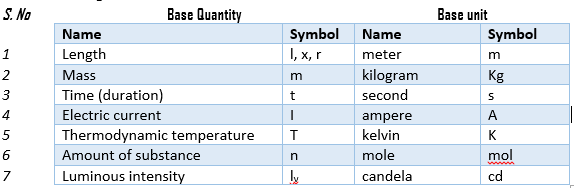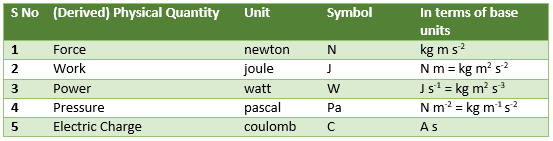## Definition of system of units

A complete set of units sufficient for the measurement of all physical quantities is called system of units.

##### Explanation
Suppose we have a set of many physical quantities; for example, mass, temperature, length, time, volume, and many more. Further suppose, we have another set of many units and this set is formed of some base units and many other derived units; for example, meter, kilogram, m3 etc. Now, if all physical quantities in the first set have a specific unit of measurement in the second set, then the second set is called the system of units for the quantities in the first set.

#### Development of the system of units

Initially, three systems of units were accepted for scientific work. However, a single unified system of units was realized to be necessary. The three systems of units were;

1. MKS system of units: Meter, Kilogram and Second were considered as base units for the measurement of length, mass and time in this system of units.
2. CGS system of units: Centimeter, Gram and Second were considered as base units for the measurement of length, mass and time, respectively.
3. Foot-Pound-Second system: In this system of units, foot, pound and second were considered as base units for the measurement of length, mass and time, respectively.

Later on, in 1960, the International Bureau on Weights and Measures agreed on a single system of units to be used in the whole world. The official name of this system is System International (SI).

## Base, derived and supplementary units

SI consists of base units, derived units and supplementary units.

### Base units

In SI, there are seven base quantities. Their units are clearly defined and are called base units. The base units are independent and do not depend on other units for their definition and understanding. The base quantities and their units are given in the following table.### Derived units

Derived units are those which are obtained by the multiplication and/or division of base units.
Some of the derived quantities and derived units are given in the following table.### Supplementary units

This class contains two units of purely geometrical quantities; radian and steradian.